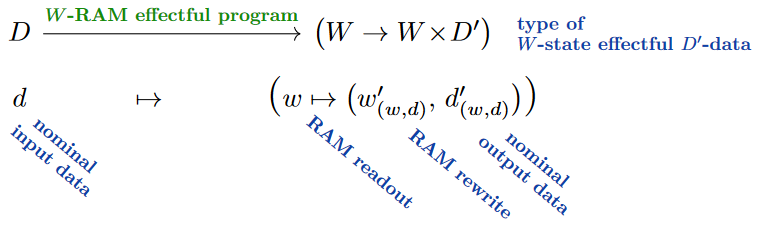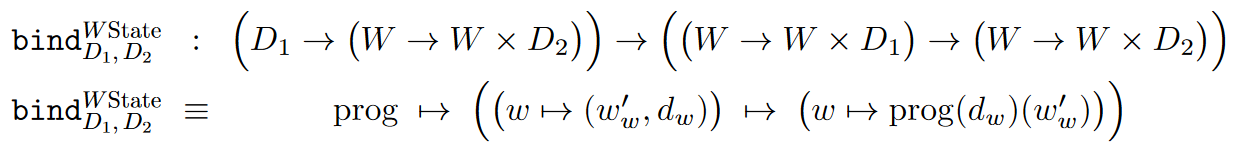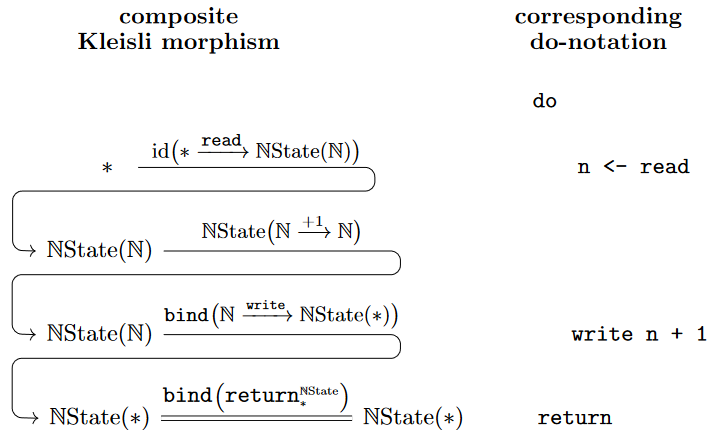Contents

Examples

# Contents

## Idea

The state monad is the name for the monad in computer science which is used to implement the functionality of read/write on a global “mutable state” (a global variable) in the context of functional programming languages.

A functional program with

1. input of (data-)type $X$,

2. output of type $Y$

3. and “mutable state” of type $W$

(e.g. the state of a “random access memory” device, cf. Yates (2019), p. 26 & Fig. 1.10)

is clearly a function (morphism) of function type between the product types with $W$ (also known as a Mealy machine, see there and cf. Oliveira & Miraldo 2016, p. 462):

$prog \;\colon\; X \times W \longrightarrow Y \times W \,.$

Now, under the hom-isomorphism of the (Cartesian product $\dashv$ internal hom)-adjunction, this is equivalently given by its adjunct, which is a function into the function type $[-,-]$ (internal hom):

$X \longrightarrow [W, W \times Y ] \,.$

Here the operation $[W, W\times (-)]$ is the monad on the type system which is induced by the above adjunction; and this latter function is naturally regarded as a morphism in the Kleisli category of this monad.

In the context of monads in computer science, this is called the state monad for mutable states of type $W$, such as for a data type $W$ of Random-Access-Memory:## Definition

Concretely, with the underlying functor being

$W State \;\colon\; D \,\mapsto\, \big[W,\, W \times D \big]$

the join operation of the $W$-state monad is given by evaluation on the intermediate $W$-variable, hence the bind operation is given as follows:To see more in detail how this encodes access to a global variable $w \colon W$ in the sense of programming languages consider the following operations which isolate the processes of reading from and writing to that variable, respectively:

$\array{ read &\colon& W State(W) \\ read &\equiv& w \mapsto (w,w) } \;\;\;\;\;\;\;\;\;\;\;\;\;\;\;\;\;\;\;\; \array{ write &\colon& W \to W State(\ast) \\ write &\equiv& w \mapsto (w' \mapsto w) }$

With these, for instance the procedure

$\array{ inc &\colon& \mathbb{N}State(\ast) \\ inc &\equiv& n \mapsto n+1 }$

which first reads-in the state of a global natural number variable and then overwrites it by its increment may be constructed as shown (on the right in do-notation) here:(This is essentially the example from Benton, Hughes & Moggi 2002 p. 68 & 71)

## Properties

### Realization in dependent type theory

In a locally Cartesian closed category/dependent type theory $\mathbf{H}$, then to every type $W$ is associated its base change adjoint triple

$\mathbf{H}_{/W} \stackrel{\overset{\sum_W}{\longrightarrow}}{\stackrel{\overset{W^\ast}{\longleftarrow}}{\underset{\prod_W}{\longrightarrow}}} \mathbf{H} \,.$

In terms of this the state monad is the composite

$State = \prod_W W^\ast \sum_W W^\ast$

of context extension followed by dependent sum, followed by context extension, followed by dependent product.

Here $\prod_W W^\ast = [W,-]$ is called the function monad or reader monad and $\sum_W W^\ast = W \times (-)$ is the coreader comonad.

Original discussion of the state/side-effect monad as a monad in computer science:

Exposition:

On the modules (“algebras”) of the state monad:

Concrete applications via implementation in Haskell:

Lecture notes:

In the generality of the local state monad:

Explicit understanding of Kleisli morphisms for the state moand as Mealy machines:

• José Nuno Oliveira, Victor Cacciari Miraldo, p. 462 in: A practical approach to state-based system calculi, Journal of Logical and Algebraic Methods in Programming 85 4 (2016) 449-474 [doi:10.1016/j.jlamp.2015.11.007]

and (almost):

• Marco Perone, Georgios Karachalias, Composable Representable Executable Machines [arXiv:2307.09090]